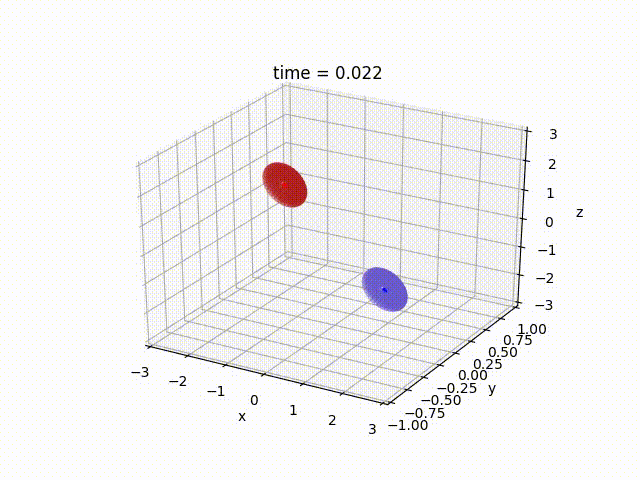Joel T. Kaardal, Ph.D.
Neural computation and machine intelligence
Introduction

Suppose there are two galaxies with massive centers (perhaps a black hole) that are each orbited by massless stellar bodies in a disc. In such a case, a galaxy would be shaped like a CD or DVD where the central hole is where the massive central body is located and the stellar bodies are located on the shiny surface that rotates rotate around the massive center (much like a disc rotates in a CD/DVD player). If these galaxies travel closely enough such that the gravity of galaxy A has a non-trivial effect on the stellar bodies of galaxy B (and vice versa), this is considered a galactic "collision".

The software is constructed in a fairly general manner to simulate a variety of different conditions. For instance, to determine the initial position and velocity of the galaxy centers only requires definition of the masses of each of the two galaxy centers, the eccentricity of the orbit (in the center-of-mass frame of reference), and the distance of closest approach (the closest distance between the two galaxy centers). The stellar bodies can be placed in arbitrary positions about the central masses (defined in the either the central mass A or B frame) and the velocities necessary for a circular orbit about the relevant central mass are calculated. Since the disc may be rotated relative to the plane in which both galaxies travel, euler angles can be defined and the software automatically rotates the stellar bodies about the relevant central body as desired. Finally, the simulation proceeds by calculating the force acting on each body and performing leapfrog integration to propagate the system forward in time.

Results

The collision of two galaxies, A and B, was simulated with equal central mass, $$M_A = M_B = \frac{5}{4}$$, and both with discs composed of rings at distances $$\left[0.2, 0.3, 0.4, \cdots, 0.7 \right]$$ (in arbitrary units) populated by $$\left[12, 18, 24, 30, 36\right]$$ stellar bodies in each rings. Both discs were rotated by an angle $$\frac{\pi}{32}$$ with respect to the collision-plane axis perpedicular to the direction of motion (see the animation). The galaxies travel along a parabolic orbit where the eccentricity is 1.0, have a closest approach distance of 0.5, and start from the phase $$\frac{2.3 \pi}{3}$$ along the Kepler orbit. Leapfrog integration was performed with a time step of dt = 0.001 from time 0.0 until 6.0 units of time passed. The results of the simlution are shown in the animation in Figure 1 where the two galaxies start off without little interaction before colliding where the retrograde rotation of the discs produces distinct tidal tails similar to what is observed in the Mice galaxy.Figure 1: the simulated collision of two disc galaxies exhibiting tidal tails.